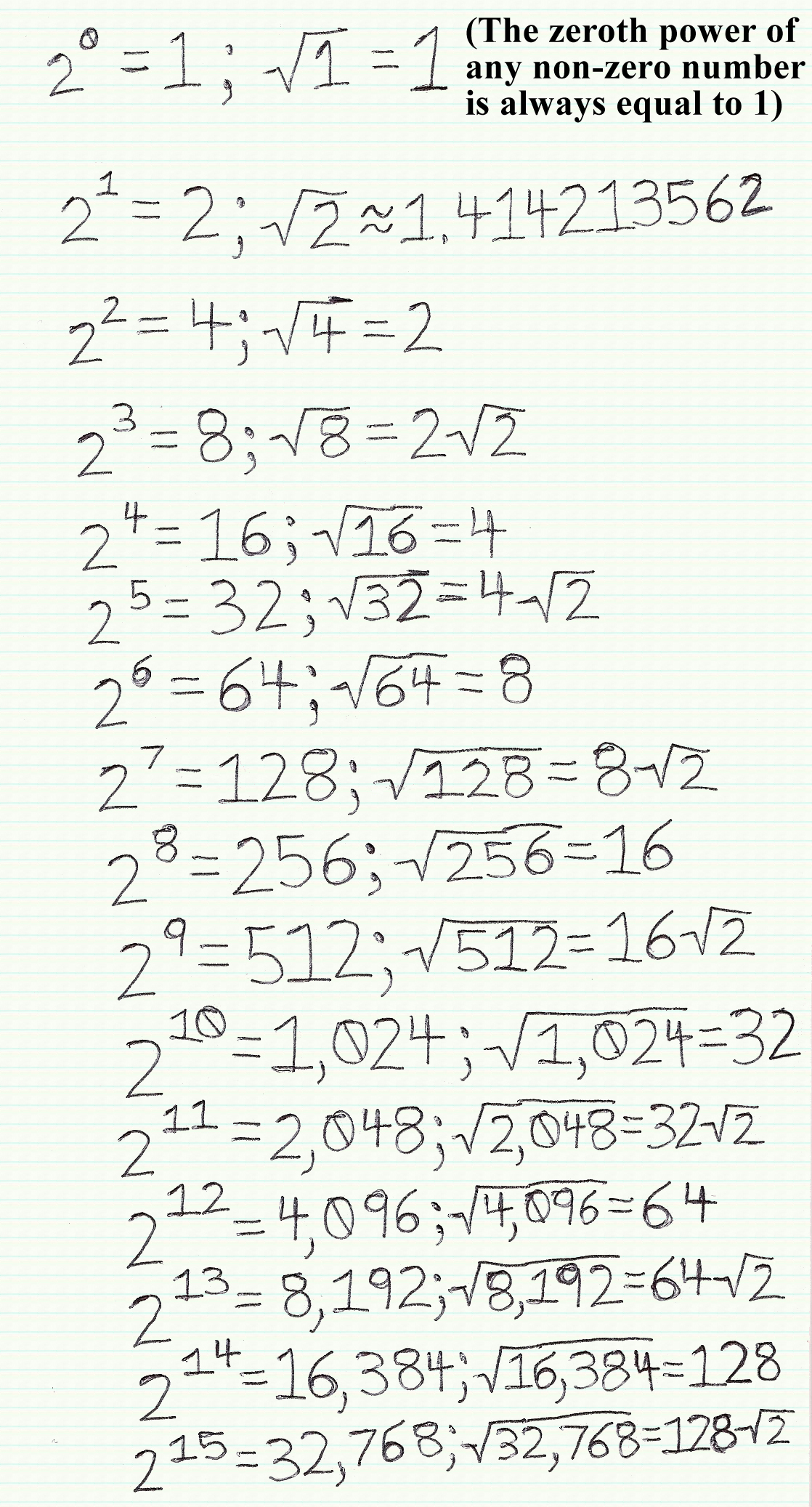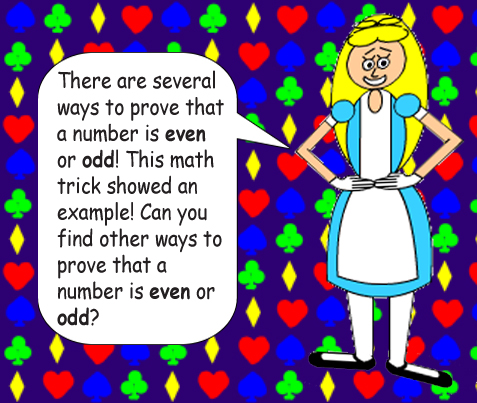# The Square Roots of the Powers of 2

## There's something special about the square roots of the powers of 2, if you look closely...Have you noticed what's special about the square roots when 2 is raised to an odd exponent? When 2 is raised to an even exponent, the square root of the power is equal to 2 to the power of the exponent's half! But when 2 is raised to an odd exponent, the square root of the power is equal to a multiple of the square root of 2! In fact, it'll be 2 to the half of the previous even exponent multiplied by the square root of 2!## X^½ = the square root of X

The caret (^) is the symbol of exponentiation. Raising a number to the power of ½ gives you the square root.

## What if you raise 2 to a negative exponent, you ask? Simple! That'll just give you the reciprocals of the positive powers!